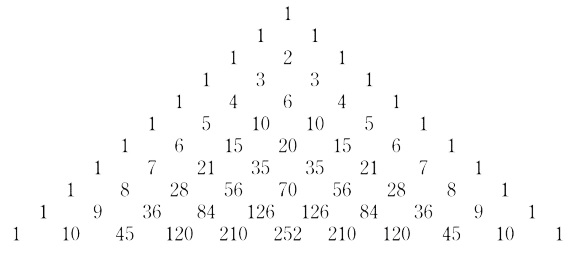# Finding the elements of nth row of Pascal's triangle in JavaScript

## Pascal's triangle:

Pascal's triangle is a triangular array constructed by summing adjacent elements in preceding rows.

The first few elements of Pascals triangle are −We are required to write a JavaScript function that takes in a positive number, say num as the only argument.

The function should return an array of all the elements that must be present in the pascal's triangle in the (num)th row.

For example −

If the input number is −

const num = 9;

Then the output should be −

const output = [1, 9, 36, 84, 126, 126, 84, 36, 9, 1];

## Example

Following is the code −

const num = 9;
const pascalRow = (num) => {
const res = []
while (res.length <= num) {
res.unshift(1);
for(let i = 1; i < res.length - 1; i++) {
res[i] += res[i + 1];
};
};
return res
};
console.log(pascalRow(num));

## Output

Following is the console output −

[
1, 9, 36, 84, 126,
126, 84, 36, 9, 1
]